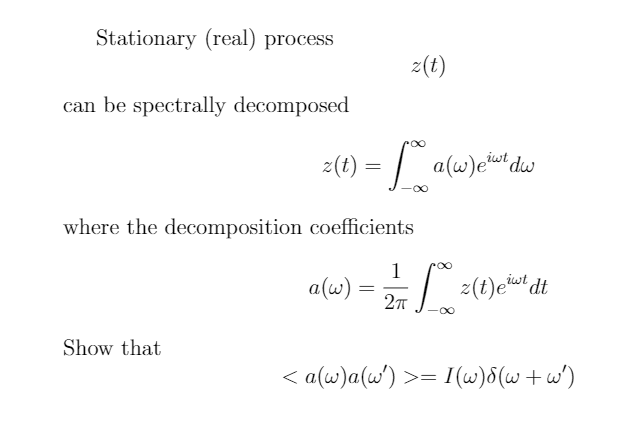# Question about the Weiner-Khinchin theorem

mrMeister
Homework Statement:
The question is attached below.
Relevant Equations:
correlation equations
Wiener-Khinchin theorem
My try:

I tried to take the expression for the decomposition coefficients and put it into the equation that I had to prove.
Then, I tried to work with the integral limits in order to get into Wiener-Khinchin theorem or maybe Fourier transform of delta function but I didn't see any success in those tries.

It would be nice to get a new point of view.

Thank you.

EDIT: I did solve this problem eventually, but I did not use the relation for z(t) - how is that?Last edited: